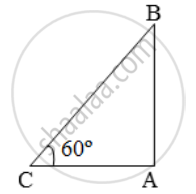# A Balloon is Connected to a Meteorological Station by a Cable of Length 200 M, Inclined at 60º to the Horizontal. Find the Height of the Balloon - Mathematics

Sum

A balloon is connected to a meteorological station by a cable of length 200 m, inclined at 60º to the horizontal. Find the height of the balloon from the ground. Assume that there is no slack in the cable

#### Solution

Let B be the balloon and AB be the vertical height. Let C be the meteorological station and CB be the cable.Then, BC = 200 m and ∠ACB = 60º

\text{Then, }\frac{AB}{BC}=\text{sin }60^\text{o}=\frac{\sqrt{3}}{2}

\Rightarrow \frac{AB}{200}=\frac{\sqrt{3}}{2}

\Rightarrow AB=( \frac{200\times \sqrt{3}}{2} \right)m=173.2m.

Concept: Heights and Distances
Is there an error in this question or solution?